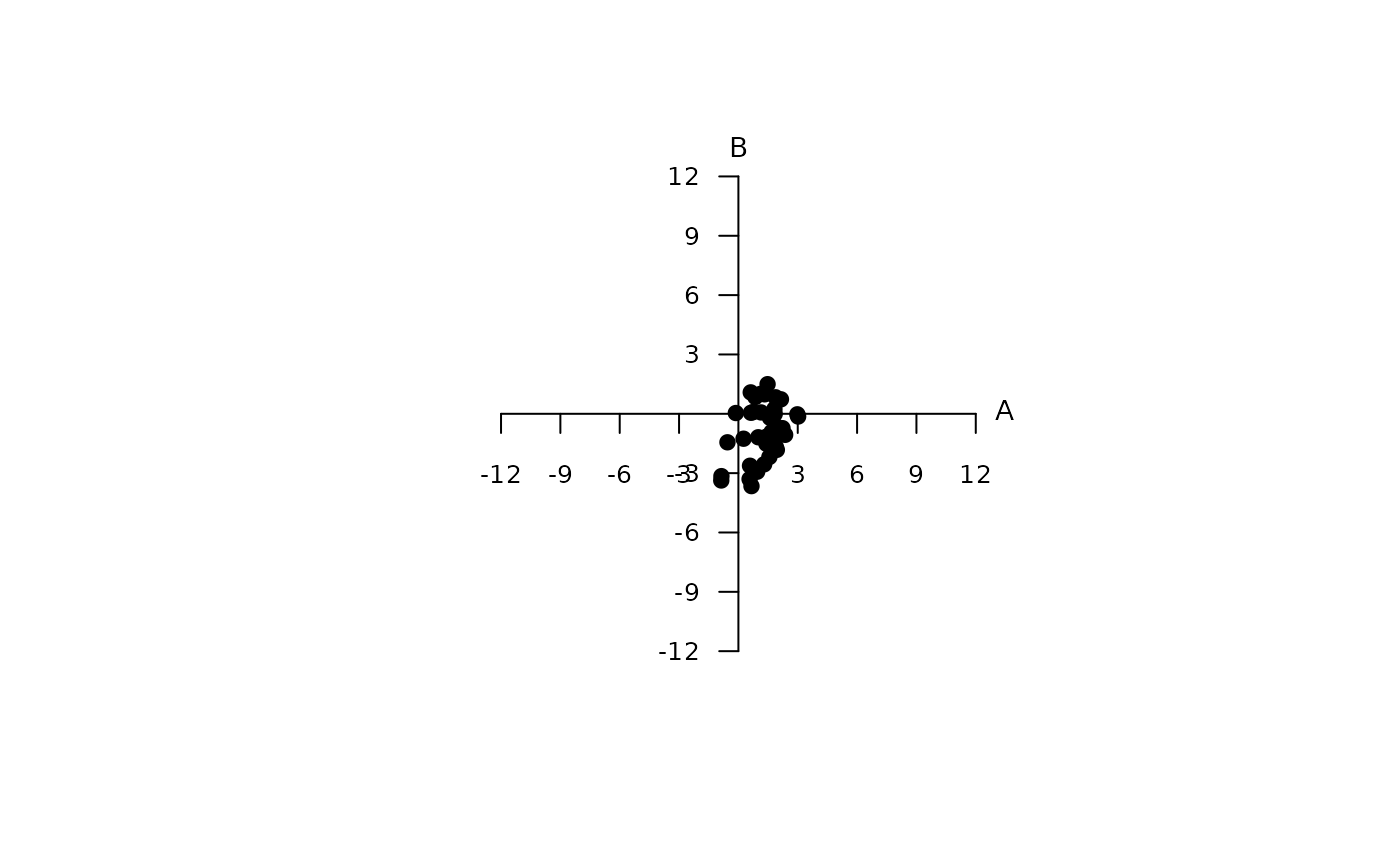Produces a plot based on the colour opponent coding diagram of Backhaus (1991).

cocplot(
cocdata,
labels = TRUE,
labels.cex = 0.9,
tick.loc = c(-12, -9, -6, -3, 3, 6, 9, 12),
achro = FALSE,
achrosize = 0.8,
achrocol = "grey",
square = TRUE,
...
)

## Arguments

cocdata

(required) a data frame, possibly a result from the colspace() or categorical() function, containing values for 'x' and 'y' coordinates as columns (labeled as such).

labels

logical. Should the name of each cone be printed next to the corresponding vertex?

labels.cex

size of the arrow labels.

tick.loc

a numeric vector specifying the location of tick marks on x & y axes.

achro

should a point be plotted at the origin (defaults to TRUE)?

achrosize

size of the point at the origin when achro = TRUE (defaults to 0.8).

achrocol

color of the point at the origin achro = TRUE (defaults to 'grey').

square

logical. Should the aspect ratio of the plot be held to 1:1? (defaults to TRUE).

...

additional graphical options. See par().

## Author

Thomas White thomas.white026@gmail.com

## Examples

data(flowers)
vis.flowers <- vismodel(flowers, visual = "apis", qcatch = "Ei", relative = FALSE, vonkries = TRUE)
coc.flowers <- colspace(vis.flowers, space = "coc")
plot(coc.flowers)